# Explain The Generation of DSB-SC Signal with Balanced Modulator Using Diodes

## Balanced Modulator Using Diodes

We  already know that a non-linear resistance or non-linear device may be used to produce Amplitude Modulation i.e. one carrier  and two sidebands .

However, a DSB-SC signal contains only two sidebands . Thus, if two non-linear devices such as diodes, transistors etc. are connected in a balanced mode so that they suppress the carriers of each other, then only sidebands are left and a  DSB-SC signal is generated .

Therefore, a balanced modulator may be defined as a circuit in which two non-linear devices are connected in a balanced mode to produce a DSB-SC signal .

In this article, we shall discuss a balanced modulator circuit using diodes .

Fig.1 shows the balanced modulator using diodes as non-linear device .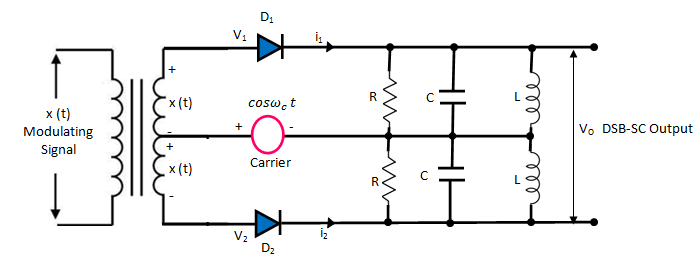Fig 1

The modulating signal x(t) is applied equally with 180phase reversal at the inputs of  both the diodes through the input center tapped transformer .

The carrier is applied to the center tap of the secondary .

Hence, input voltage to Dis given by :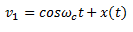……..(1)

And the input voltage to Dis given by :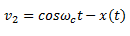……….(2)

The parallel RLC circuits on the output side form the band pass filters .

### Analysis

The diode current iand i2 are given by :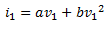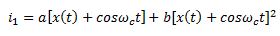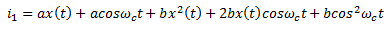………………(3)

Similarly,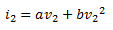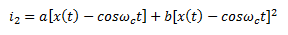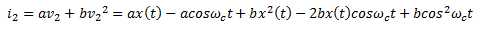…………(4)

The output voltage is given by :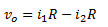Substituting the expression for iand i2 from equations (3) and (4), we get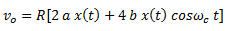Or,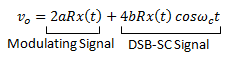Hence, the output voltage contains a modulating signal term and the DSB-SC signal .

The modulating signal term is eliminated and the second term is allowed to pass through to the output by the LC band pass filter section .

Therefore, final output = 4 b R x(t) cos ωct

= K x(t) cos ωct

Thus, the diode balanced modulator produces the DSB-SC signal at its output .